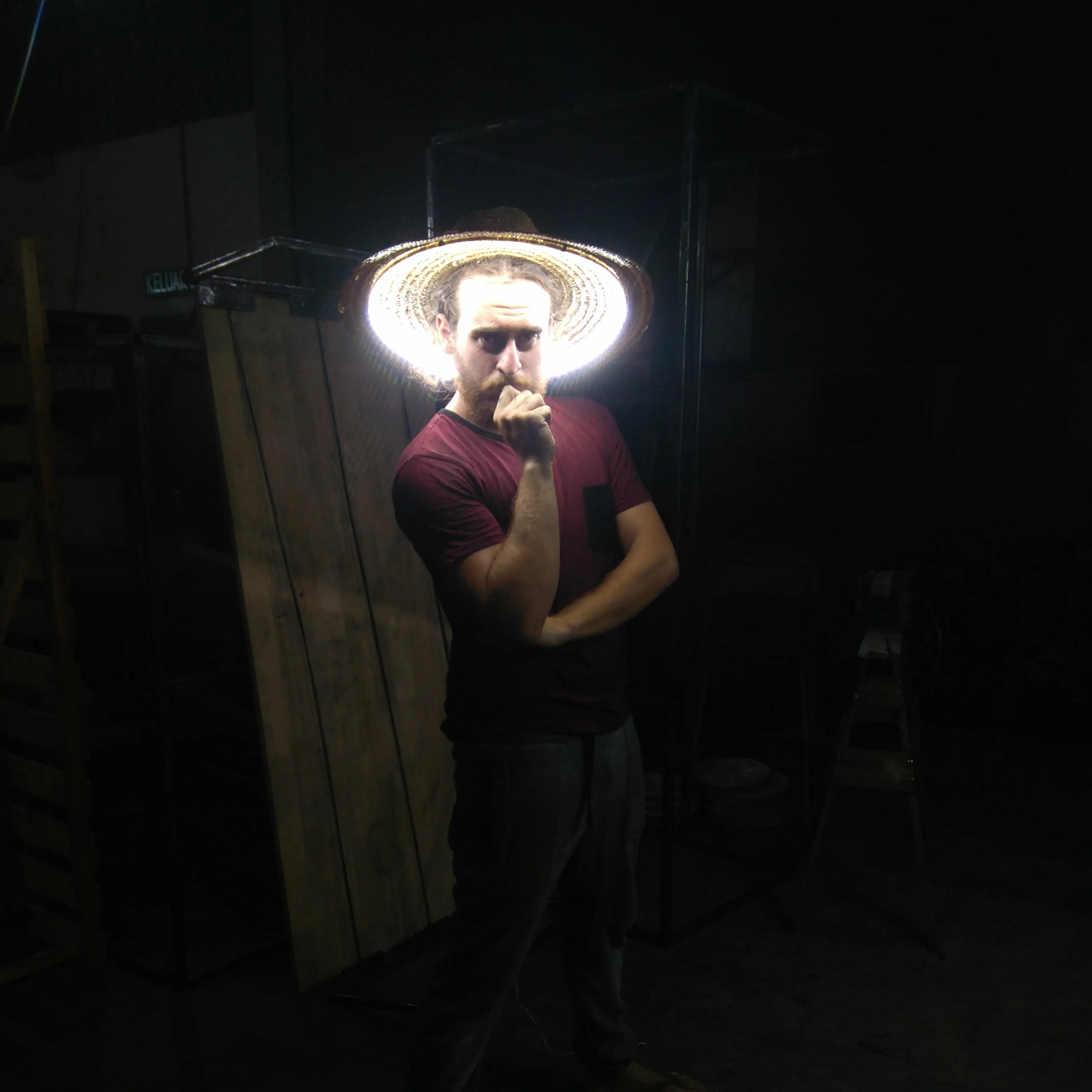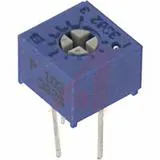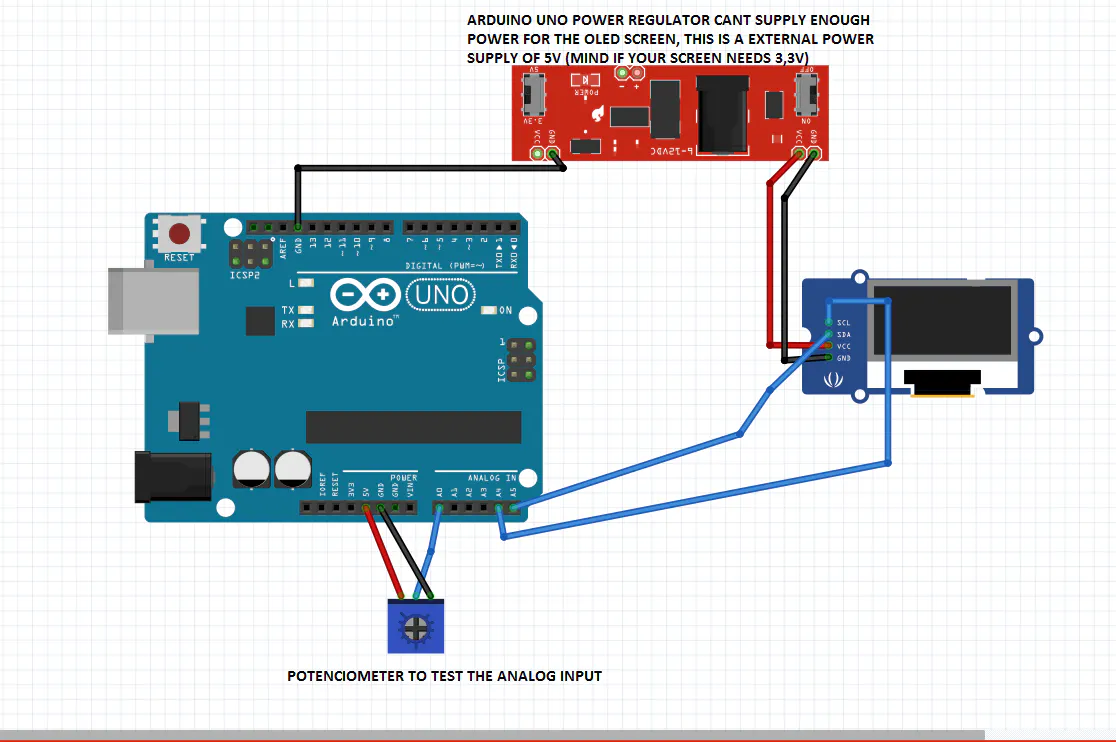# 2€ OLED I2C Analog Pin Dynamic Plotting

Cool project to begin with 128x64 OLED, done with a twisted logic to fix the refresh flashing screen.

BeginnerShowcase (no instructions)1 hour7,676

## Things used in this project

### Hardware componentsArduino UNO & Genuino UNO it could be done with nano with enough memory (atmega328)
×1
×1Jumper wires (generic)
×1Single Turn Potentiometer- 10k ohms FOR TESTING
×1
 EXTERNAL POWER 5V for the oled, arduino is not enough
×1×1

### Software apps and online servicesArduino IDE

## Schematics

### OLED SCHEMATIC

:)## Code

### OLED code, initial text and analog read

C/C++
```///coded by Javier Muoz Sez with the help of https://github.com/klauscam/Arduino-Cellular-Automata/blob/master/CellularAutomata.ino
//any question ,suggestion, joboffer, or singing telegram adress to javimusama@gmail.com
//enjoy

#include <SPI.h>
#include <Wire.h>

#define OLED_RESET 4
#define retardo 60
#define escalaejey 10 //en pixels
int i=0,j=0,ii=0,iii=0,muevepantalla=0;
uint8_t arraydatos;
uint8_t arrayauxiliar;

void setup()   {
display.begin(SSD1306_SWITCHCAPVCC, 0x3C); //initialize with the I2C addr 0x3C (128x64)
display.clearDisplay();
display.display();

//comment below part to erase the text or put in your own
display.setCursor(10,22);
display.setTextSize(1);
display.setTextColor(WHITE);
display.println(F("Javi es to pepino"));
////////////////////////////////////////////////////

pinMode(A0,INPUT);

//initialice data array with all 0
for(i=0;i<=129;i++)
{arraydatos[i]=0;}
i=0;
for(i=0;i<=129;i++)
{arrayauxiliar[i]=0;}
i=0;
}

void loop() {
grid();//SET A FANCY GRID

//THIS FUNCTION DOES THE MAGIC
for(iii=0;iii<=128;iii++)
{

if(arraydatos[iii+1]<=arraydatos[iii])//IF OLD DATA IS GREATER THAN NEW DATA STORES 1 IN ARRAYAUXILIAR
{arrayauxiliar[iii]=1;}
if(arraydatos[iii]==0){arrayauxiliar[iii]=0;}//IF NOT MAKE SURE THAT 0 IS STORED IN ARRAYAUXILIAR
if(arraydatos[iii+1]==arraydatos[iii])
{arrayauxiliar[iii]=0;}
arraydatos[iii]=arraydatos[iii+1];//COPY THE DATA ONE POSITION TO THE LEFT
}

arraydatos=map(analogRead(A0),0,1023,0,63);//NEW DATA READ FROM ANALOG PIN STORED AT THE END OF THE ARRAY

plotdata();
}

void grid ()//EASY FUNCTION , DRAW A WHITE DOT MULTIPLE TIMES MAKING A GRID
{
for(ii=0;ii<=43;ii++)
{
for(i=0;i<=7;i++)
{
display.drawPixel(ii*3,escalaejey*i,WHITE);
}

}
display.display();
}

void plotdata()//THIS DOES SOME MAGIC TOO
{
for(i=0;i<=126;i++)
{
if(arrayauxiliar[i]==1){display.drawFastVLine(i,0,63-arraydatos[i],BLACK);}//IF NEW DATA VALUE IS SMALLER THAN OLD DATA VALUE WE NEED TO BUILD THE DATA SECTOR BY SWITCHINGOFF pixels which otherwise would remain ON.
if(arrayauxiliar[i]==0){display.drawFastVLine(i,63-arraydatos[i],arraydatos[i],WHITE);} //IF NOT JUST SWITCH ON THE PIXELS CREATING A VerticalLine
}
display.display();

}
```

## Credits

### javier muñoz sáez

10 projects • 48 followers
Electronic engineer and sparky person. I make tutorials so my future self doesnt need to remember where he put the code